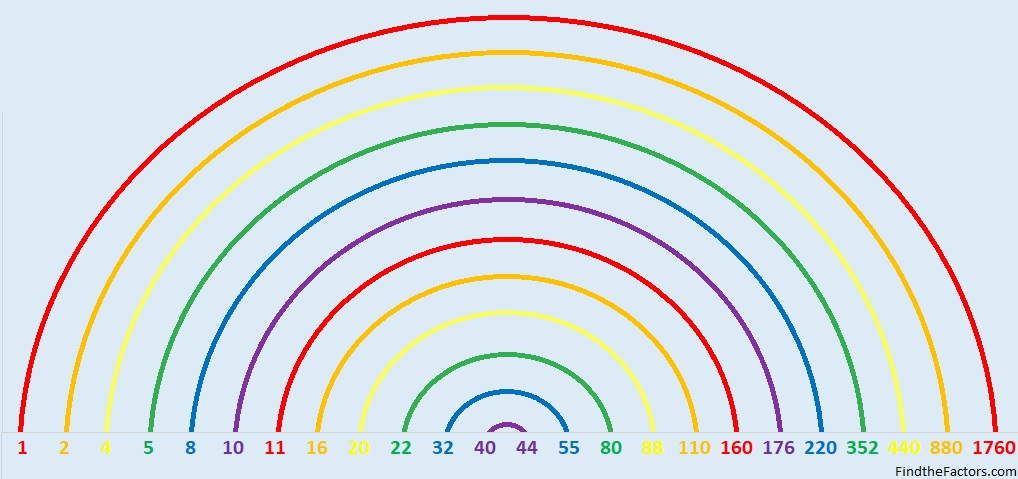# 1760 Pots of Gold and Rainbows

Contents

### Today’s Puzzle:

Write the numbers 1 to 12 in the first column and again in the top row so that those numbers are the factors that make the given clues. It’s a level 6, so it won’t be easy. Finding a leprechaun’s pot of gold isn’t easy either. Still, if you can solve this puzzle, then you will have found some real golden nuggets of knowledge.They say there’s a pot of gold at the end of the rainbow. Where’s the rainbow?

### Factors of 1760:

Puzzle number, 1760, has many factors. It makes a very big factor rainbow!• 1760 is a composite number.
• Prime factorization: 1760 = 2 × 2 × 2 × 2 × 2 × 5 × 11, which can be written 1760 = 2⁵ × 5 × 11.
• 1760 has at least one exponent greater than 1 in its prime factorization so √1760 can be simplified. Taking the factor pair from the factor pair table below with the largest square number factor, we get √1760 = (√16)(√110) = 4√110.
• The exponents in the prime factorization are 5, 1, and 1. Adding one to each exponent and multiplying we get (5 + 1)(1 + 1)(1 + 1) = 6 × 2 × 2 = 24. Therefore 1760 has exactly 24 factors.
• The factors of 1760 are outlined with their factor pair partners in the graphic below.### More About the Number 1760:

1760 is the hypotenuse of a Pythagorean triple:
1056-1408-1760 which is (3-4-5) times 352.

1760 is the difference of two squares in eight different ways:
441² – 439² = 1760,
222² – 218² = 1760,
114² – 106² = 1760,
93² – 83² = 1760,
63² – 47² = 1760,
54² – 34² = 1760,
51² – 29² = 1760,  and
42² – 2² = 1760. (That means we are only four numbers away from the next perfect square!)

1760 is palindrome 2102012 in base 3
because 2(3⁶)+1(3⁵)+ 0(3⁴)+2(3³)+0(3²)+1(3¹)+2(3º) = 1760.

This site uses Akismet to reduce spam. Learn how your comment data is processed.